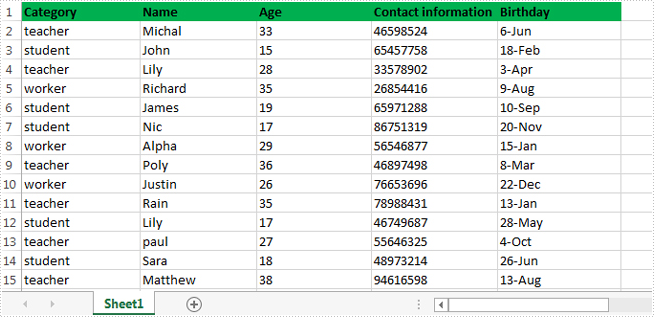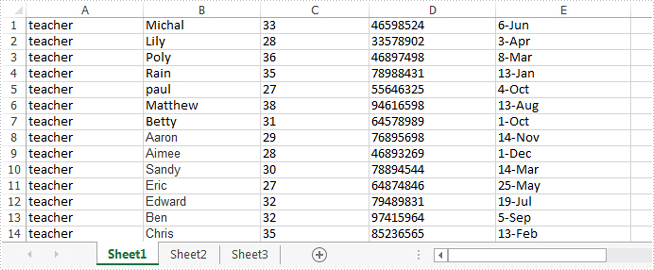# Retrieve data from one excel worksheet and extract to a new excel file in C#

Searching data is a powerful data processing function of Microsoft excel, but it doesn't allow users to extract the selected data to a new excel file directly. It's almost impossible for us to copy data row by row manually from one excel file to another, so it cannot entirely meet our requirements especially when we want to retrieve and extract the interesting data from a large excel file.

This article will demonstrate how to retrieve data from one excel worksheet and extract to a new excel file with Spire.XLS in C#.

Below is the screenshot of the original excel worksheet:In this sample, all of the data related to teacher were extracted to a new excel file.

Detail steps overview:

Step 1: Create a new workbook instance and get the first worksheet.

```Workbook newBook = new Workbook();
Worksheet newSheet = newBook.Worksheets;
```

Step 2: Create a new workbook instance and load the sample excel file.

```Workbook workbook = new Workbook();
```

Step 3: Get the worksheet where you want to retrieve and extract data from. In this sample, it's the first worksheet.

```Worksheet sheet = workbook.Worksheets;
```

Step 4: Retrieve data and extract to the first worksheet of the new excel workbook.

```int i = 1;
int columnCount = sheet.Columns.Count();
foreach (CellRange range in sheet.Columns)
{
if (range.Text == "teacher")
{
CellRange sourceRange = sheet.Range[range.Row, 1, range.Row, columnCount];
CellRange destRange = newSheet.Range[i, 1, i, columnCount];
sheet.Copy(sourceRange, destRange, true);
i++;
}
}
```

Step 5: Save the target file as NewForm.xlsx.

```newBook.SaveToFile("NewForm.xlsx", ExcelVersion.Version2010);
```

Effective screenshot:Full codes:

```using System.Linq;
using Spire.Xls;

namespace Retrieve_and_extract_data
{
class Program
{
static void Main(string[] args)
{
Workbook newBook = new Workbook();
Worksheet newSheet = newBook.Worksheets;
Workbook workbook = new Workbook();
Worksheet sheet = workbook.Worksheets;
int i = 1;
int columnCount = sheet.Columns.Count();
foreach (CellRange range in sheet.Columns)
{
if (range.Text == "teacher")
{
CellRange sourceRange = sheet.Range[range.Row, 1, range.Row, columnCount];
CellRange destRange = newSheet.Range[i, 1, i, columnCount];
sheet.Copy(sourceRange, destRange, true);
i++;
}
}
newBook.SaveToFile("NewForm.xlsx", ExcelVersion.Version2010);

}
}
}
```